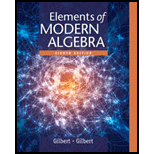# In the 27 -letter alphabet A described in Example 2 , use the affine cipher with key a = 7 and b = 5 to encipher the following message. all systems go What is the inverse mapping that will decipher the ciphertext? Example 2 Translation Cipher Associate the n letters of the "alphabet" with the integers 0 , 1 , 2 , 3 ..... n − 1 . Let A = { 0 , 1 , 2 , 3 ..... n - 1 } and define the mapping f : A → A by f ( x ) = x + k m o d n where k is the key , the number of positions from the plaintext to the ciphertext. If our alphabet consists of a through z , in natural order, followed by a blank, then we have 27 "letters" that we associate with the integers 0 , 1 , 2 , ... , 26 as follows: Alphabet: a b c d e f ... v w x y z "blank" A: 0 1 2 3 4 5 21 22 23 24 25 26### Elements Of Modern Algebra

8th Edition
Gilbert + 2 others
Publisher: Cengage Learning,
ISBN: 9781285463230### Elements Of Modern Algebra

8th Edition
Gilbert + 2 others
Publisher: Cengage Learning,
ISBN: 9781285463230

#### Solutions

Chapter
Section
Chapter 2.8, Problem 5E
Textbook Problem

## Expert Solution

### Want to see the full answer?

Check out a sample textbook solution.See solution

### Want to see this answer and more?

Bartleby provides explanations to thousands of textbook problems written by our experts, many with advanced degrees!

See solution